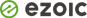In Pandas, we can only plot values with the numeric data type. If you try to plot with any other Data Type other than numeric data, Python will raise TypeError: no numeric data to plot.

In this article, we will see what exactly “TypeError: no numeric data to plot” means and how to resolve this with examples.

## What is TypeError: no numeric data to plot?

The error occurs mainly when you attempt to plot values from pandas DataFrame, but it turns out there are no numeric values present in DataFrame.

The common mistake developers make is to assume that a certain column in the DataFrame is numeric, but actually, it will be of a different type.

Let us take a simple example to reproduce this issue.

In the below example, we have cricket teams, and we will create a line plot for the columns points, runrate, and wins using Pandas DataFrame.

``````# import pandas library
import pandas as pd
import matplotlib.pyplot as plt

# create pandas DataFrame
df = pd.DataFrame({'team': ['India', 'South Africa', 'New Zealand', 'England'],
'points': ['10', '8', '3', '5'],
'runrate': ['0.5', '1.4', '2', '-0.6'],
'wins': ['5', '4', '2', '2']})

# print the data frame
print(df)
df[['points', 'runrate', 'wins']].plot()
plt.show()``````

Output

``TypeError: no numeric data to plot``

When you run the program, Python will raise TypeError: no numeric data to plot.

If you look at the code at first glance, all the columns represent numeric values. However, if you check the type of each column, you will see that it is of type object.

We can check the data type of each column using the `dtpyes `function.

`print(df.dtypes)`
``````# Displays the Data Type of each column in Pandas DataFrame
print(df.dtypes)

# Output
team       object
points     object
runrate    object
wins       object
dtype: object
``````

If you look at the output, none of the columns in the DataFrame are numeric, and it is of type object.

## How to fix TypeError: no numeric data to plot?

We can resolve the TypeError by converting the data to be plotted into numeric data.

There are 2 methods available to convert the data into numeric values while plotting the DataFrame columns.

### Method 1: Using DataFrame.astype() function

The `DataFrame.astype()` method is used to cast the Pandas object into a specific data type.

Syntax:

`df['column_name']= df['column_name'].astype(data_type)`

Let us resolve the issue by converting the Pandas object to numeric dtype using `astype()` function.

``````# import pandas library
import pandas as pd
import matplotlib.pyplot as plt

# create pandas DataFrame
df = pd.DataFrame({'team': ['India', 'South Africa', 'New Zealand', 'England'],
'points': ['10', '8', '3', '5'],
'runrate': ['0.5', '1.4', '2', '-0.6'],
'wins': ['5', '4', '2', '2']})

# print the data frame
print(df)

# convert the columns to numeric using astype() function
df['points']=df['points'].astype(float)
df['runrate']=df['runrate'].astype(float)
df['wins']=df['wins'].astype(float)

df[['points', 'runrate', 'wins']].plot()
plt.show()``````

Output

We are able to plot the lines successfully as the columns are converted to numeric values. We can check the dtypes once again by using `dtypes` function.

``````# Displays the Data Type of each column in Pandas DataFrame
print(df.dtypes)

# Output
team        object
points     float64
runrate    float64
wins       float64
dtype: object``````

### Method 2 :Using pandas.to_numeric() function

The `pandas.to_numeric()` function is used to convert the argument to a numeric type.

The default return dtype is float64 or int64, depending on the data supplied. We can use the downcast parameter to obtain other dtypes.

Syntax:

`df['column_name'] = pd.to_numeric(df['column_name'])`

Let us resolve the issue by converting the Pandas object to numeric dtype using `to_numeric()` function.

``````# import pandas library
import pandas as pd
import matplotlib.pyplot as plt

# create pandas DataFrame
df = pd.DataFrame({'team': ['India', 'South Africa', 'New Zealand', 'England'],
'points': ['10', '8', '3', '5'],
'runrate': ['0.5', '1.4', '2', '-0.6'],
'wins': ['5', '4', '2', '2']})

# print the data frame
print(df)

# convert the columns to numeric using to_numeric() function
df['points']=pd.to_numeric(df['points'])
df['runrate']=pd.to_numeric(df['runrate'])
df['wins']=pd.to_numeric(df['wins'])

print(df.dtypes)
df[['points', 'runrate', 'wins']].plot()
plt.show()``````

Output

We are able to plot the lines successfully as the columns are converted to numeric values (int and float). We can check the dtypes once again by using `dtypes` function.``````# Displays the Data Type of each column in Pandas DataFrame
print(df.dtypes)

# Output
team        object
points       int64
runrate    float64
wins         int64
dtype: object
``````

## Conclusion

The TypeError: no numeric data to plot occurs mainly when you attempt to plot values from pandas DataFrame, but it turns out there are no numeric values present in DataFrame.

We can resolve the TypeError by converting the data to be plotted into numeric data. There are 2 methods available to convert the data into numeric values while plotting the DataFrame columns.

• Convert to numeric using `DataFrame.astype()` function
• Convert to numeric using `pandas.to_numeric()` function